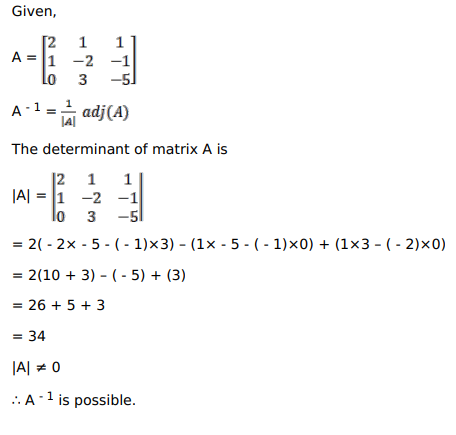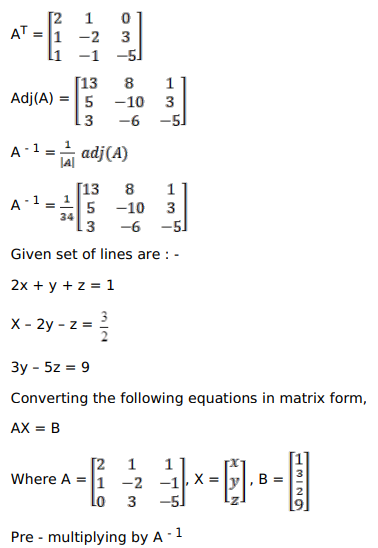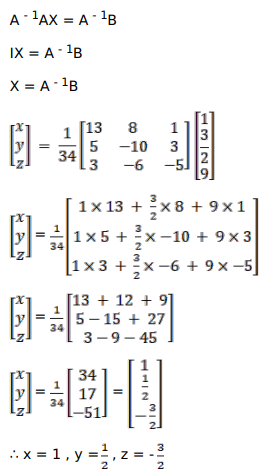# Solve this following

Question:

If $A=\left(\begin{array}{ccc}2 & 1 & 1 \\ 1 & -2 & -1 \\ 0 & 3 & -5\end{array}\right)$, find $A^{-1}$

Using $A^{-1}$, solve the following system of linear equations:

$2 x+y+z=1$

$x-2 y-z=\frac{3}{2}$

$3 y-5 z=9$

HINT: Here $A=\left(\begin{array}{ccc}2 & 1 & 1 \\ 1 & -2 & -1 \\ 0 & 3 & -5\end{array}\right)$,

$\mathbf{X}=\left(\begin{array}{l}x \\ y \\ z\end{array}\right)$ and $\mathbf{B}=\left(\begin{array}{l}1 \\ 3 / 2 \\ 9\end{array}\right)$

Solution: